# How to Type Math

• Latex code looks like a bunch of unintelligible gibberish when you look at it, but over time, you might grow to love it! Latex allows you to write math seamlessly into your English text without leaving your keyboard! Plus, it's $$\textcolor{purple}{\text{ pretty}}.$$ And it's powerful. Learn to write Latex, and you'll be a happier math person!

# How to get into math mode:

Typing the double backslash "\\", typing the double dollar-sign "  " and clicking the arrow-X in the menu above all put you into math mode. Just remember that whichever you choose to open your math with should be the same thing that you use to close your math with.

### The "%" math mode

Clicking on the "%" icon above the post editor will automatically give you the math mode wrapper of "'''"s.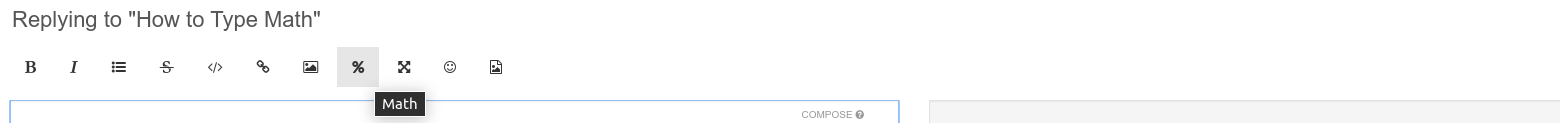The wrapper comes with a very fancy sophisticated code example, highlighted in $$\textcolor{blue}{\text{ blue }}.$$ Go ahead and delete this, because you are about to put in your own fancy sophisticated code.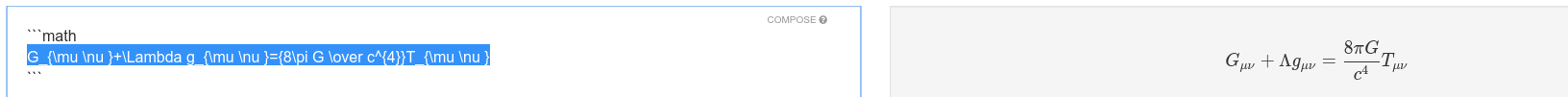Type your code directly inside this wrapper. Please keep in mind that to make a new line, typing "enter" won't automatically do it for you. You will have to type two backslashes at the end of each line.

3 \times 4 \\
x \times y \times y \\
0.5 \times 1.\overline{111}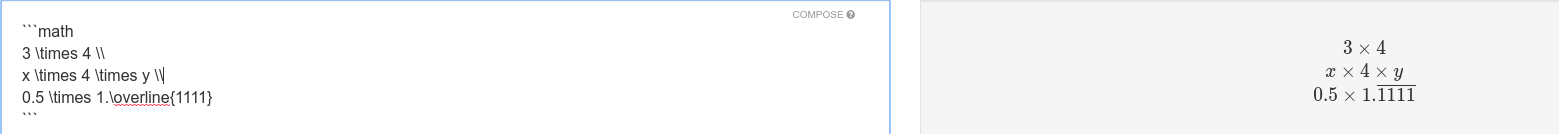## The backslash method

I personally like to use the backslashes because I find it easier to keep my hands on the keyboard without having to go for the mouse. With this method, you must type in the "wrapper" yourself. This is two backslashes, followed by an open parentheses / close parentheses. If you use curvy brackets, you get inline Latex that can sit inside your sentence.

There are random math \$$x^2\$$ symbols \$$2^2\$$ appearing \$$\frac{1}{2}\$$ in this \$$x^y\$$ sentence.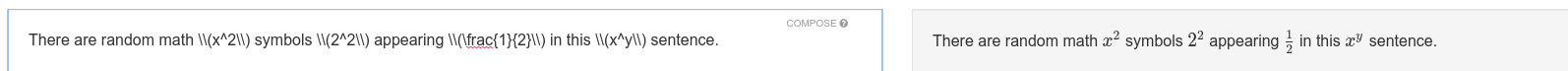If you use square brackets, you get Latex on its own line.

There are random math $x^2$ symbols $2^2$ appearing $frac{1}{2}$ in this $x^y$ sentence.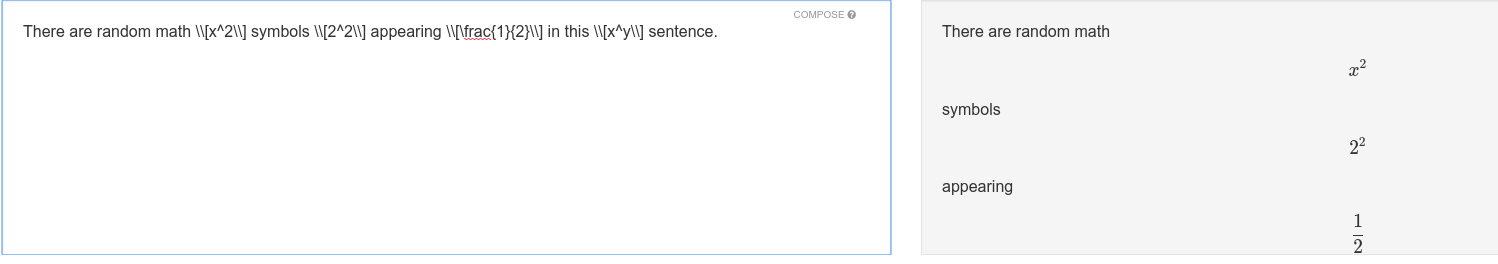## The Dollar-Sign Approach

Typing two dollar signs will also open and close your Latex code. However, it gives e x t r a h u g e spacing, and will set the math on its own line.

3 \times 4
x \times 4 \times y
0.5 \times 1.\overline{1111}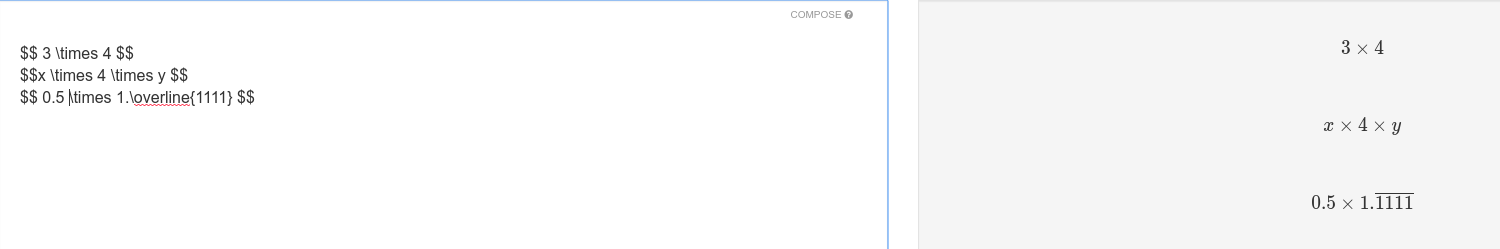# Latex Examples

3 \times 4
x \times 4 \times y
0.5 \times 1.\overline{1111}


With wrapper: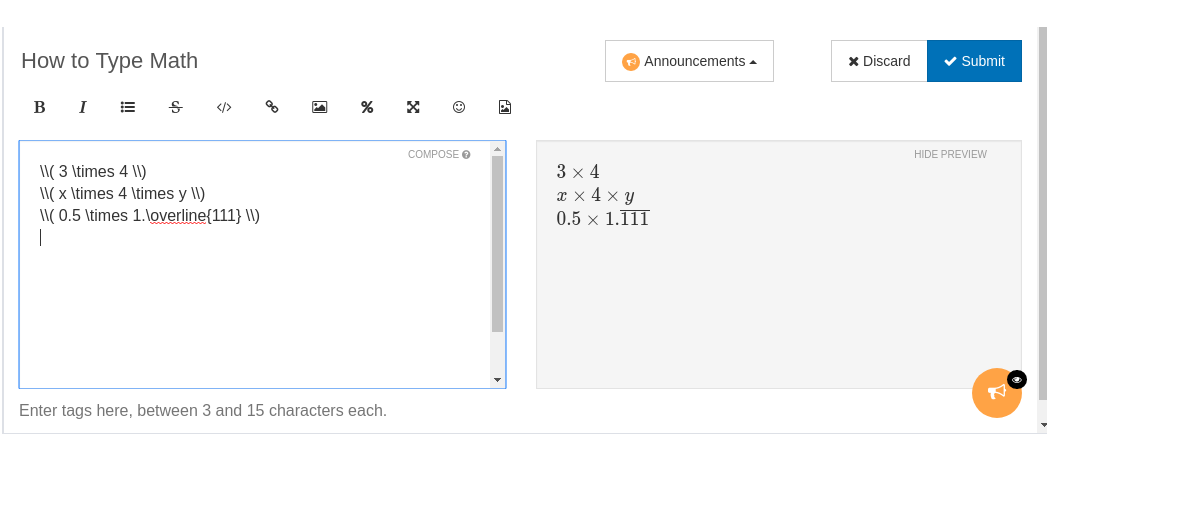3 + 2 = 5
3 + 2 \neq 4
3 + 2 > 5
3 + 2 \geq 4
2 + 2 \leq 5

2 + 2 = 4
3 + 2 > 4


With wrapper: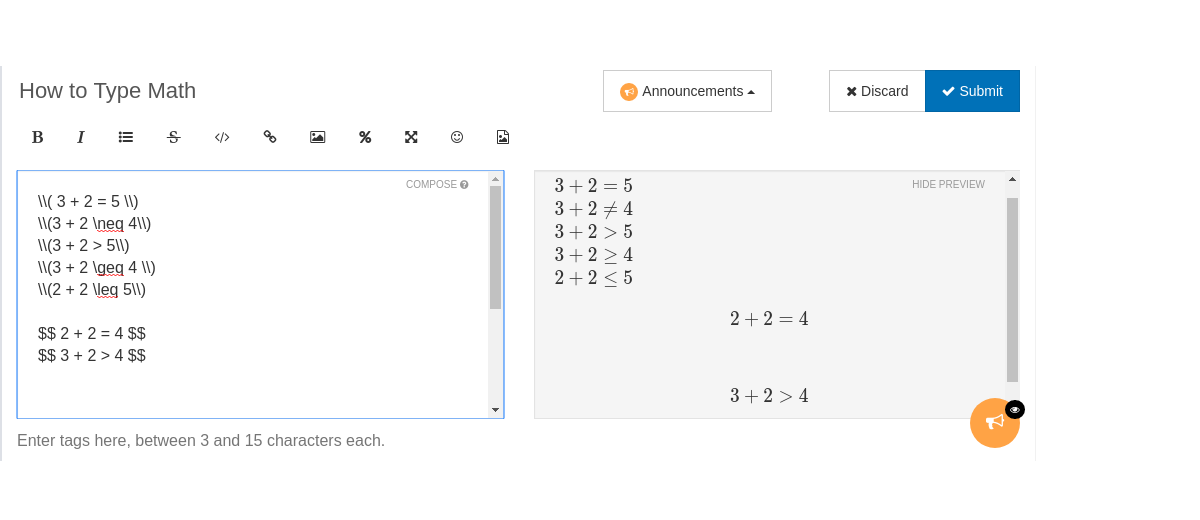When typing fractions, it helps to first type the empty braces, like this:

\frac{}{}


before you enter the values into the braces.

\frac{123456}{7890}


This way, you're less likely to make a typo!

 \frac{1}{2}
\frac{1}{2 + x}
\frac{\frac{1}{2}}{x}
\frac{1}{2} \times \frac{x}{3}
\frac{1}{2} = x


With wrapper: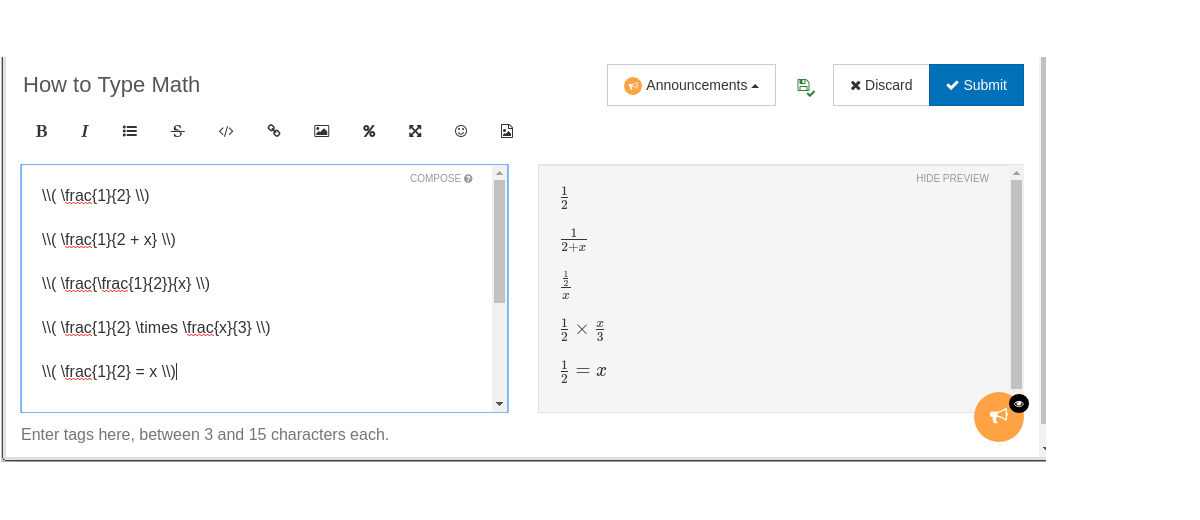Superscripts, subscripts and degree notation. Remember that if the superscript or subscript is more than one digit, you need braces { } around it.

x^2
x^{30}
360^{\circ}
x_1, x_2, x_3, ldots
x_{20}



With wrapper: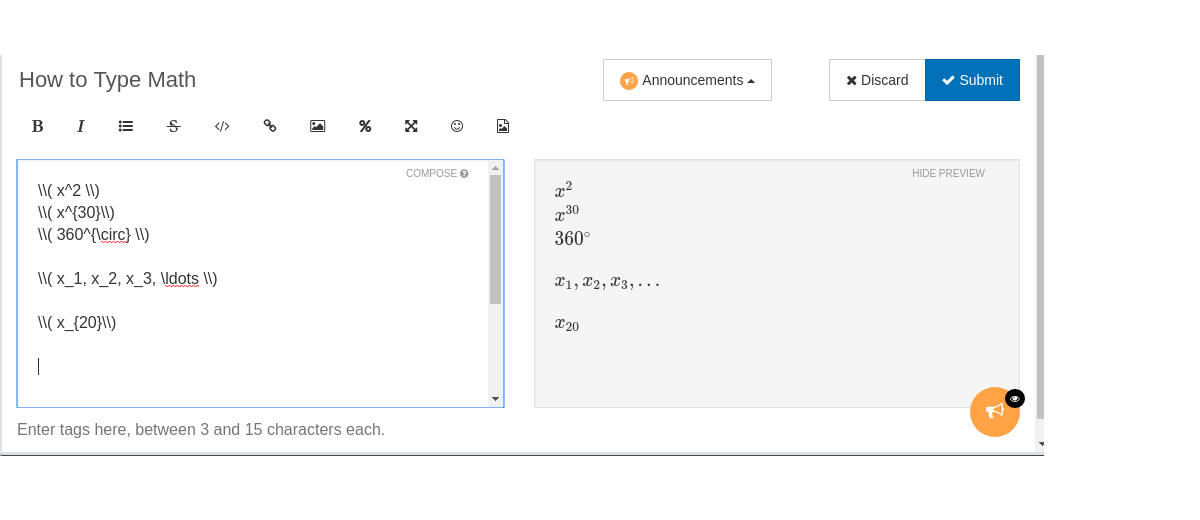## Geometric symbols:

 \angle ABC
\bigtriangleup ABC
\overline{AB} = 3
x \approx 3
\angle A = 30^{\circ}
\frac{ \overline{AB}}{\overline{BC}} = 2


With wrapper: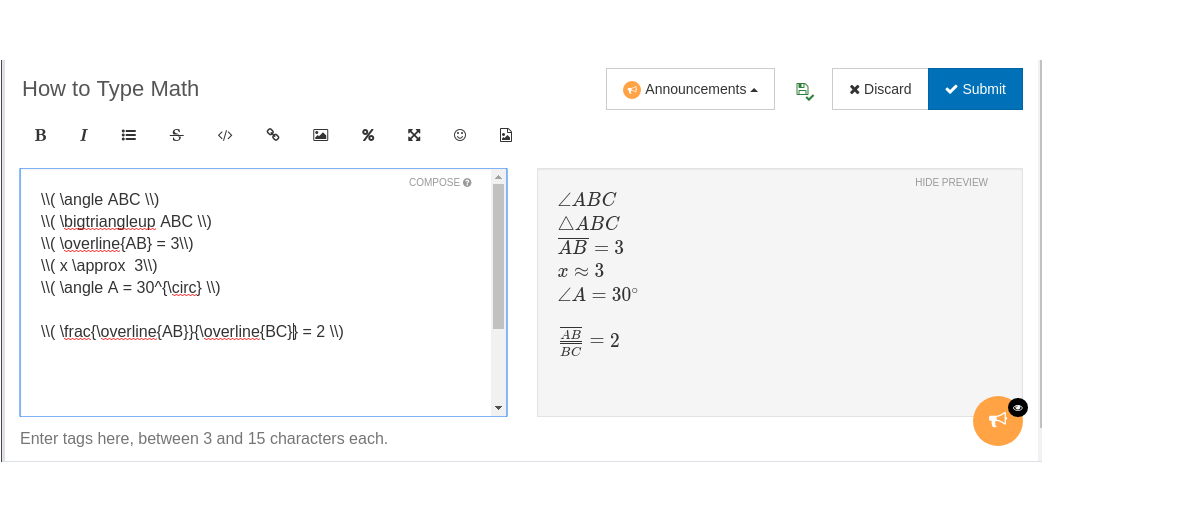Aligned equation blocks:

\begin{aligned}
3x + 2 &= 7 \\
6x + 4 &= 14 \\
x &= \frac{5}{3}
\end{aligned}


With wrapper: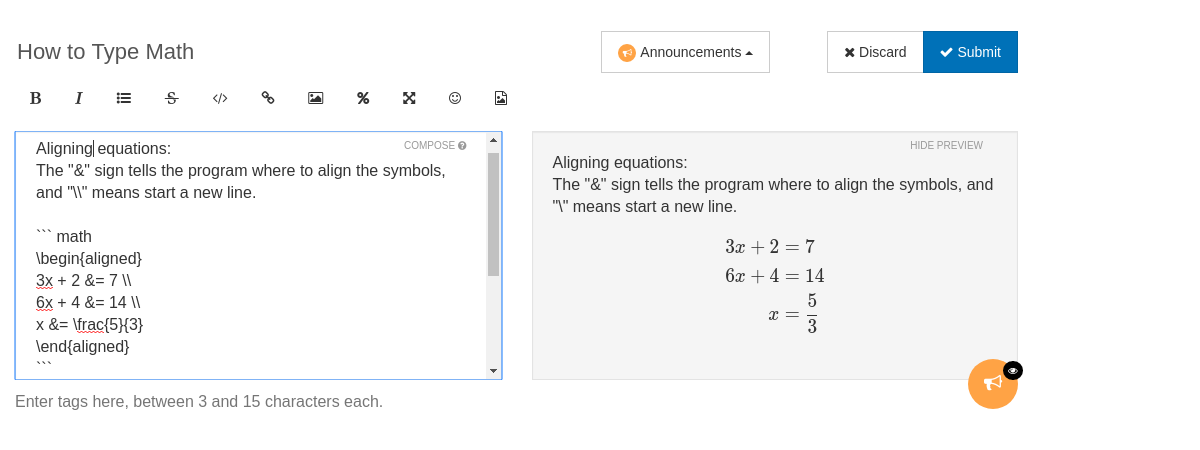• This post is deleted!

• When you want to type a $or % symbol in Latex, you must put two backslashes before it: \\$ 40
50 \\%
\\# 5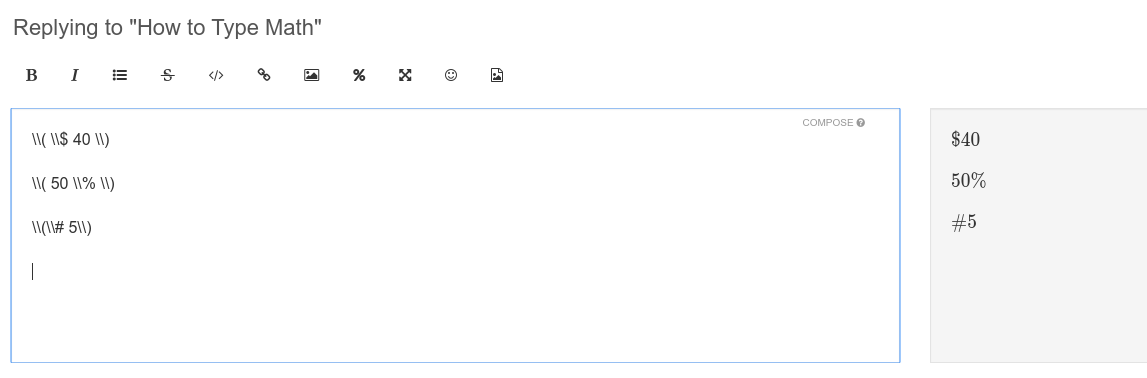• Additionally, to make colored text, you can use the "color{colorname}" command within math mode. Everything you enter until the end of the math mode will render in the color name entered as colorname.

math
\color{red} \text{Latex does not regard spaces:} 1 2 3 4 5 6
\color{blue} Text written without the \text{ \text{command} } formatting looks squished like this
\color{green} \text{ A green equation: } \frac{a + b}{c} + 2.5 - x^2
\color{purple} \text{ Purple text using the command 'text'} \\)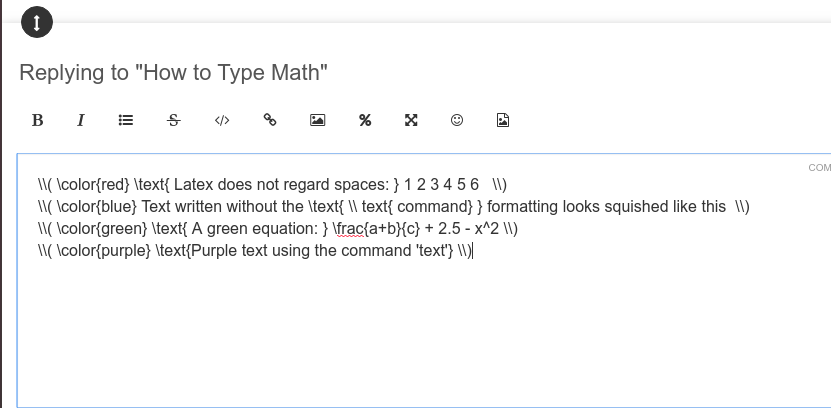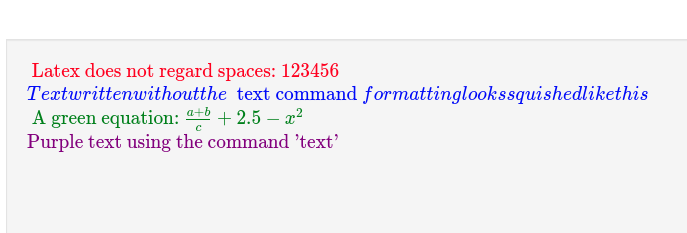•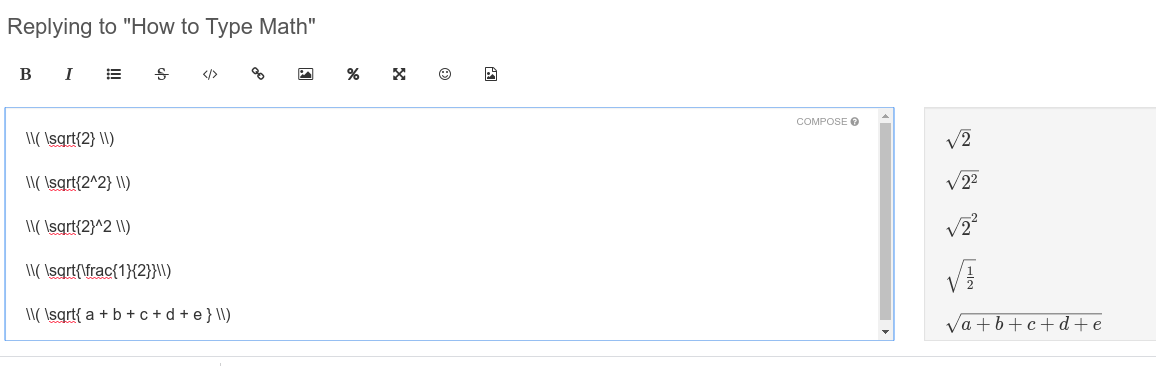• $$\color{lightgray} \text{This post is deleted!}$$

• $$\color{lightgray}\text{This post is deleted!}$$

• $$\footnotesize\color{lightgray} \text{This post is deleted!}$$

• $$\small\color{lightgray} \text{This post is deleted!}$$
Hmmm.... How do you change the font?

• @divinedolphin I'd suggest searching for "Markdown change font." Markdown is the language that this forum uses, and it's a shorthand form of html code. I don't know how to do this myself, sorry!I hope you have luck finding the answer!

• $$\color{gray} \text{This post is}$$ $$\color{black} \text{not}$$ $$\color{gray} \text{deleted.}$$
Lol• Does the sigma function work here?

• @The-Rogue-Blade

Thanks for asking! You can type the Sigma $$\sum$$ by itself like this:

\$$\sum \$$


or as the sum of a sequence of terms, like this:

\$$\sum_{n=1}^{n=10} n^2 \$$


$$\sum_{n=1}^{n=10} n^2$$

\$$\sum_{n=1}^{\infty} n^2 \$$


$$\sum_{n=1}^{\infty} n^2$$

I hope this helps!• [\LaTeX]

[\text{L}^\text{A}\text{T}_\text{E}\text{X}]

[L^AT_EX]

• @divinedolphin How come that post still shows up as code? When I edit it the preview shows up fine, but this is what I see right now.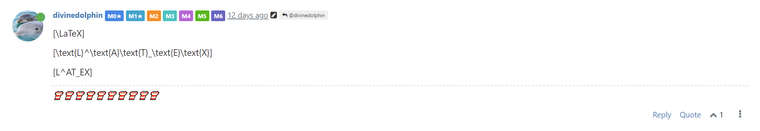• @divinedolphin Try this instead:

$$LaTeX$$

$$\text{L}^\text{A}\text{T}_\text{E}\text{X}$$

\$$LaTeX\$$

\$$\text{L}^\text{A}\text{T}_\text{E}\text{X}\$$•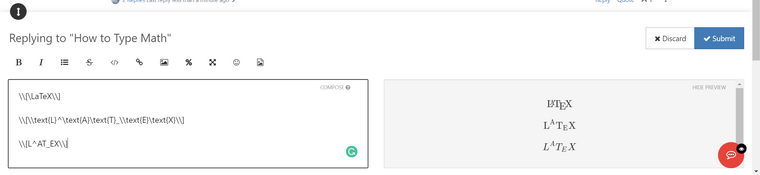• @divinedolphin Yes, I've noticed that too; the square brackets don't seem to render. I usually use the double backslash with the round brackets.• $$\LaTeX$$
$$\text{L}^\text{A}\text{T}_\text{E}\text{X}$$
$$L^AT_EX$$

• @divinedolphin Yay, you did it!